Examples

Chapter 4 Class 12 Determinants
Serial order wise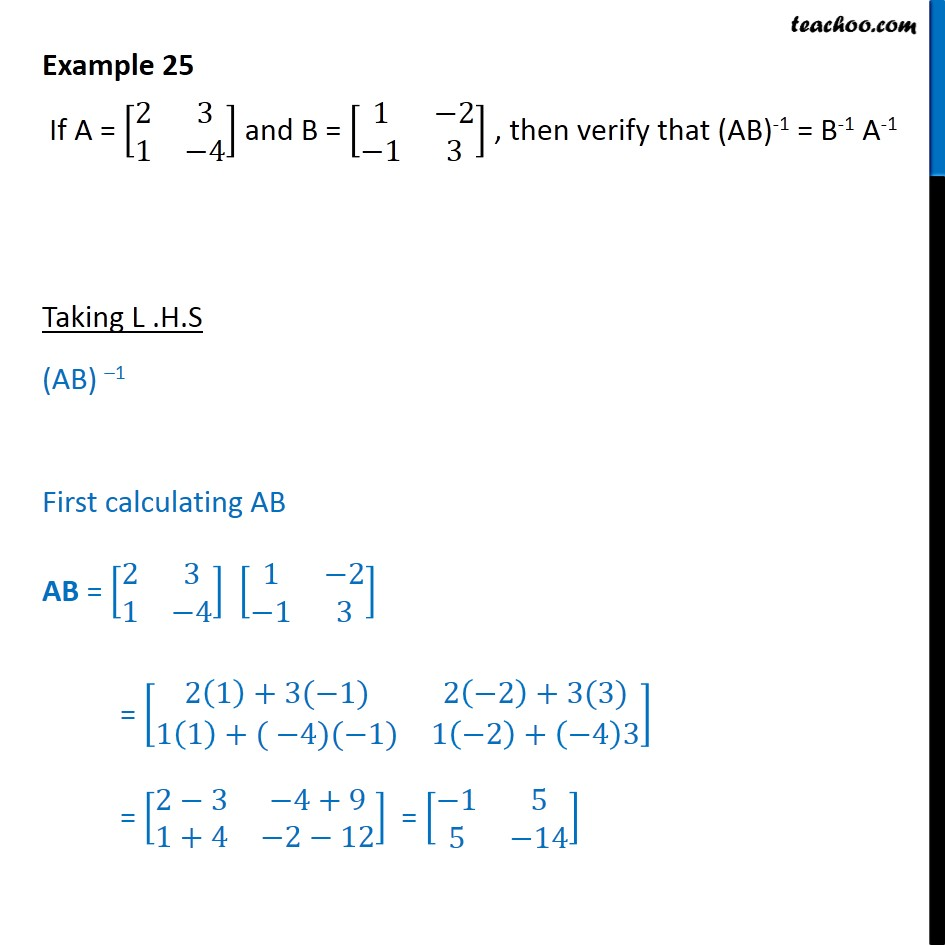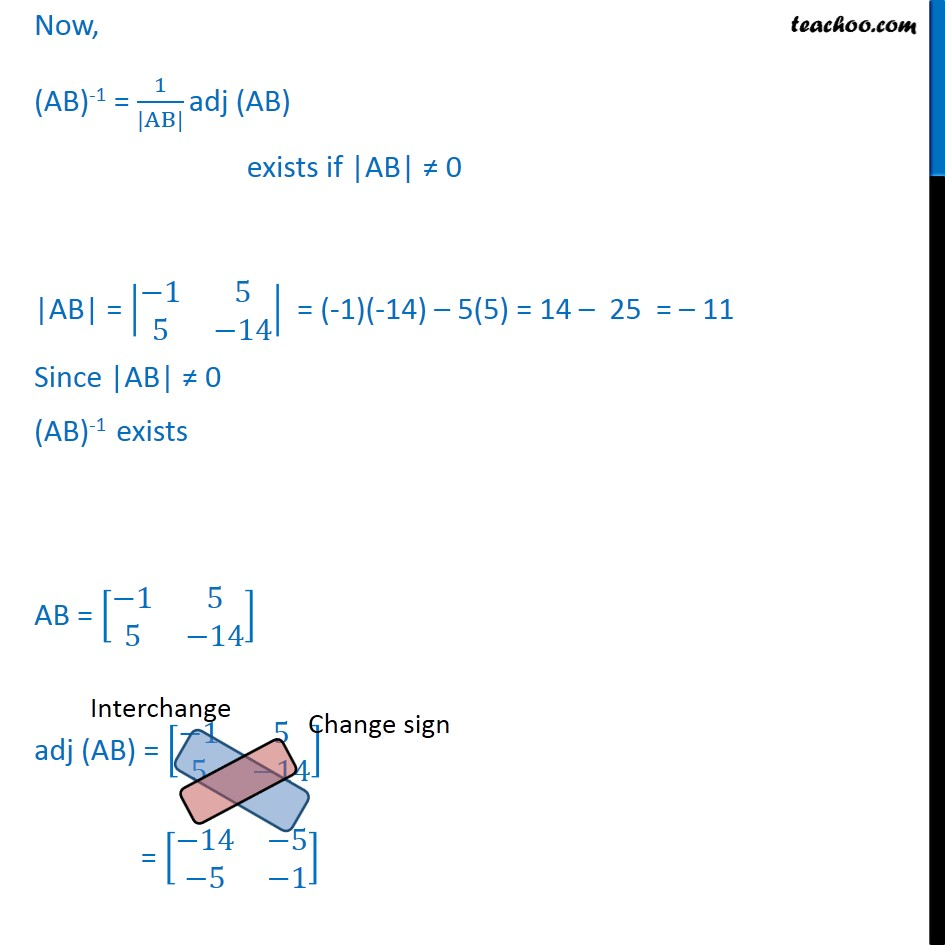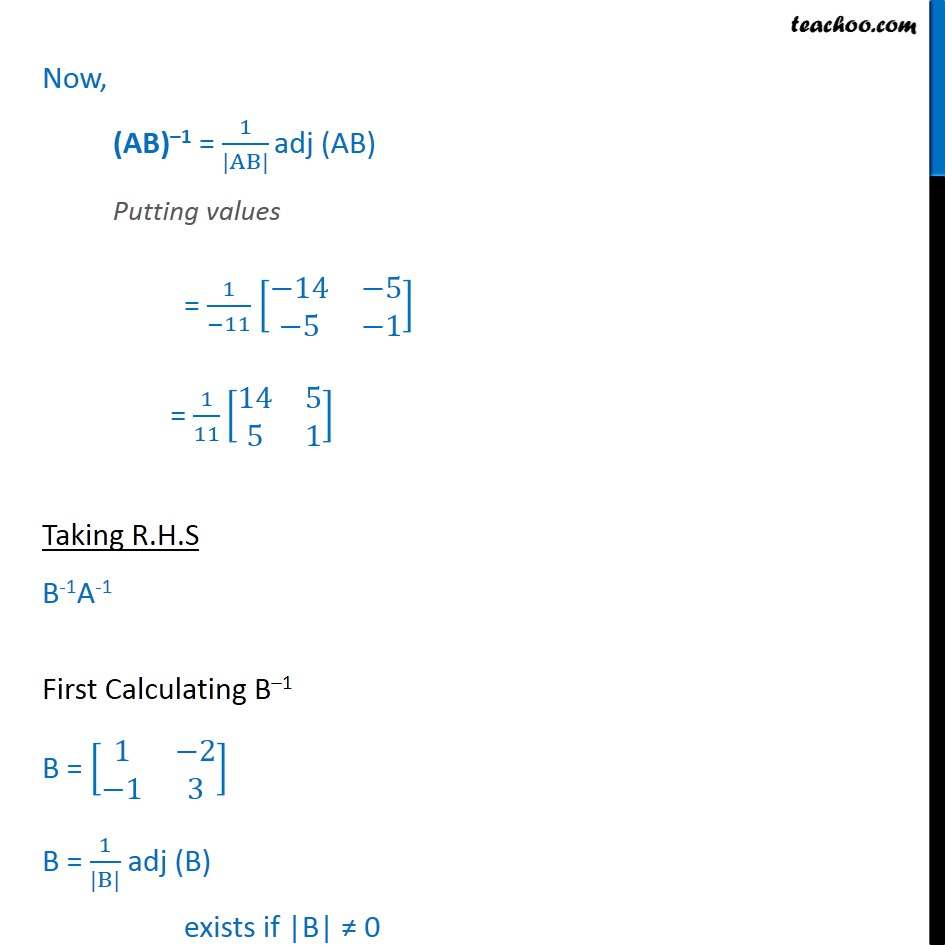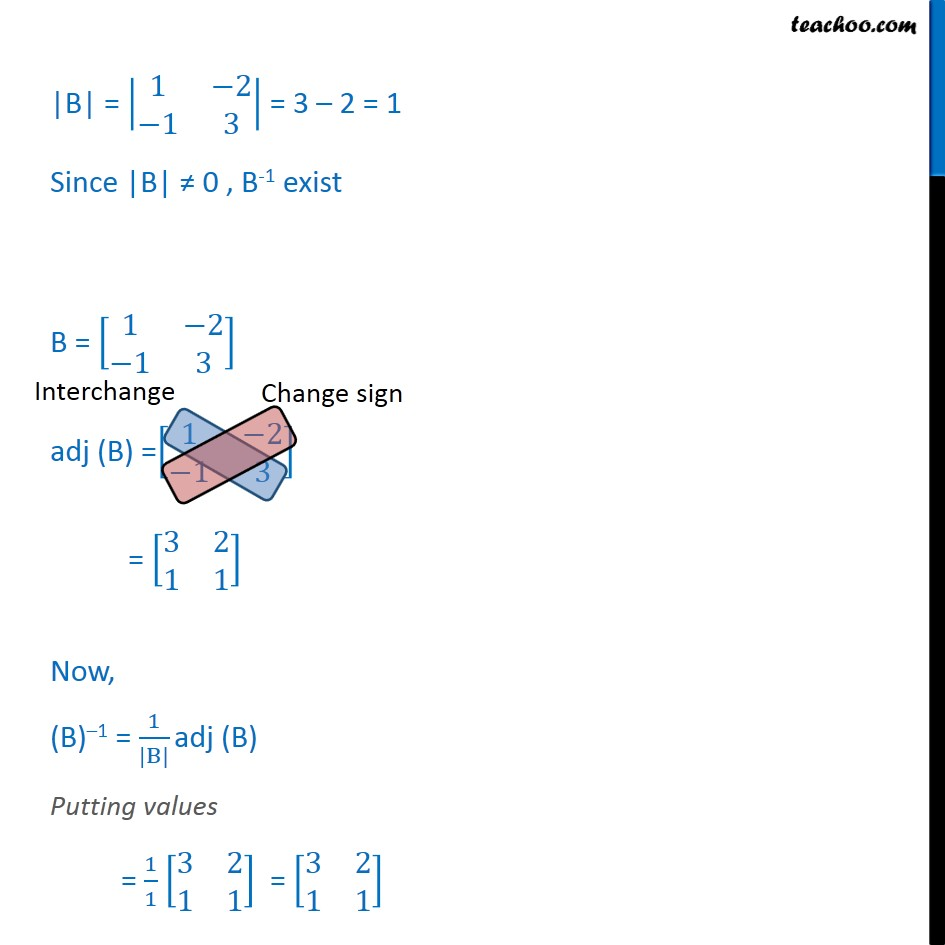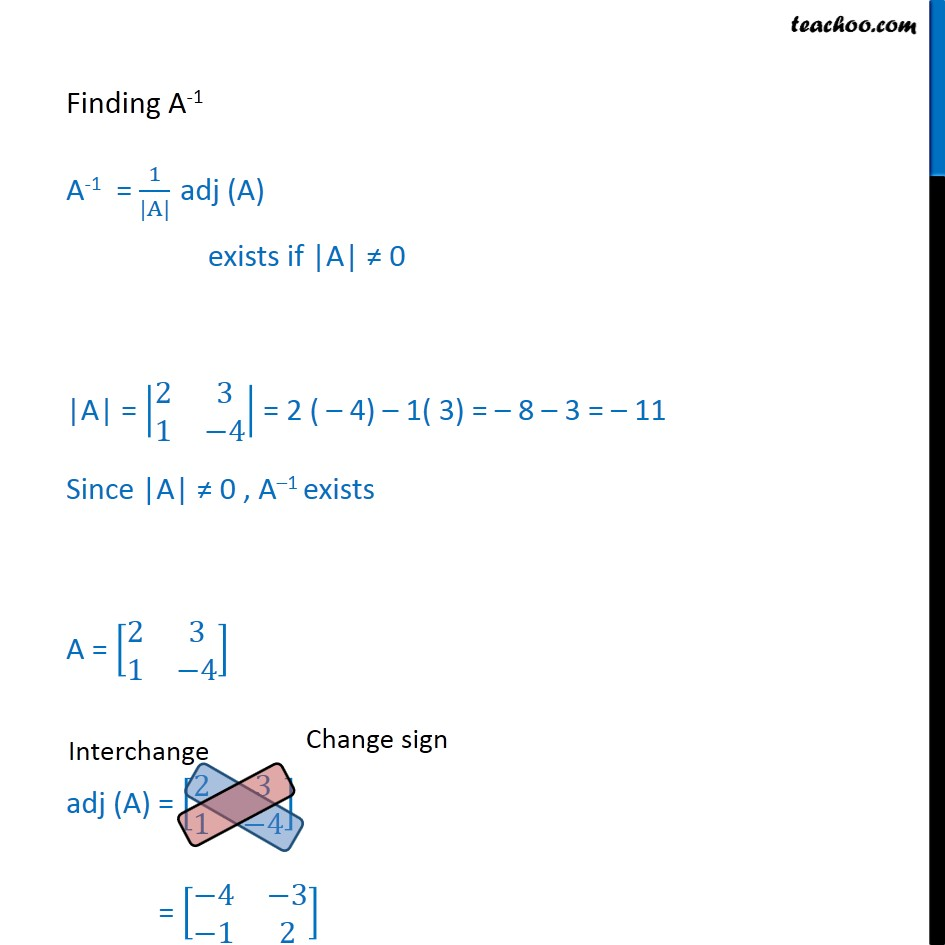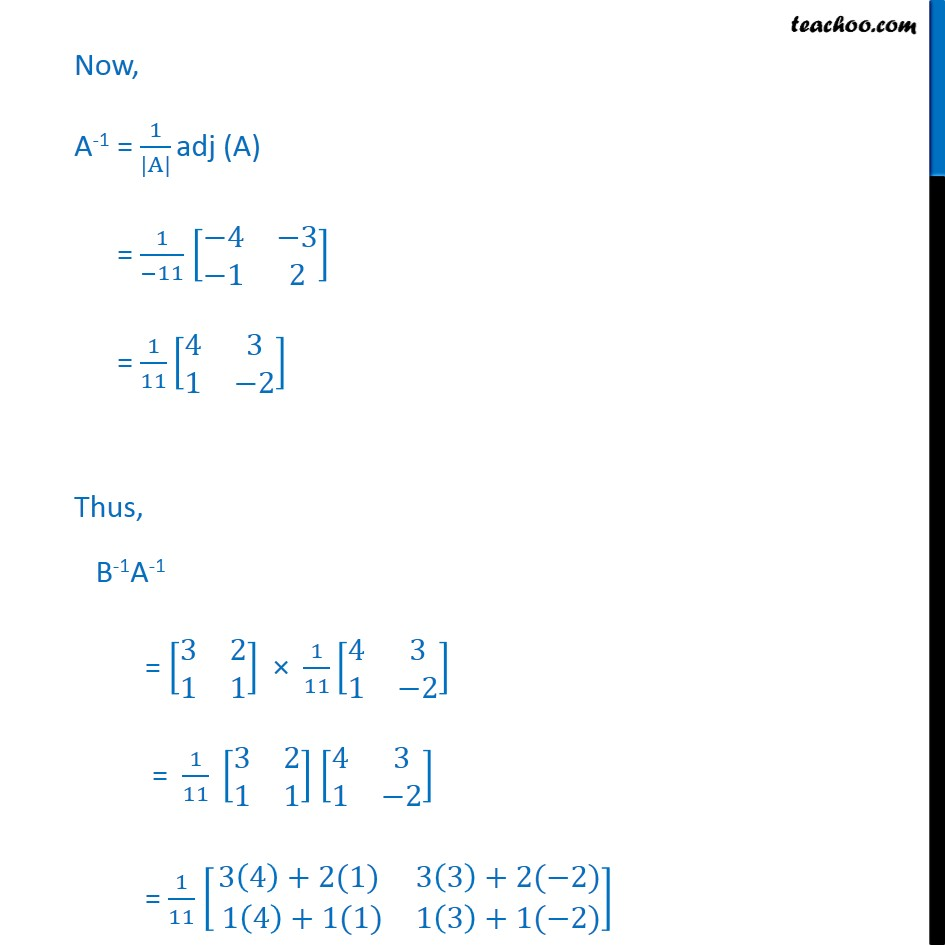Get live Maths 1-on-1 Classs - Class 6 to 12

### Transcript

Example 25 If A = 2 3 1 4 and B = 1 2 1 3 , then verify that (AB)-1 = B-1 A-1 Taking L .H.S (AB) 1 First calculating AB AB = 2 3 1 4 1 2 1 3 = 2 1 +3( 1) 2 2 +3(3) 1 1 +( 4)( 1) 1 2 + 4 3 = 2 3 4+9 1+4 2 12 = 1 5 5 14 Now, (AB)-1 = 1 |AB| adj (AB) exists if |AB| 0 |AB| = 1 5 5 14 = (-1)(-14) 5(5) = 14 25 = 11 Since |AB| 0 (AB)-1 exists AB = 1 5 5 14 adj (AB) = 1 5 5 14 = 14 5 5 1 Now, (AB) 1 = 1 |AB| adj (AB) Putting values = 1 11 14 5 5 1 = 1 11 14 5 5 1 Taking R.H.S B-1A-1 First Calculating B 1 B = 1 2 1 3 B = 1 |B| adj (B) exists if |B| 0 |B| = 1 2 1 3 = 3 2 = 1 Since |B| 0 , B-1 exist B = 1 2 1 3 adj (B) = 1 2 1 3 = 3 2 1 1 Now, (B) 1 = 1 |B| adj (B) Putting values = 1 1 3 2 1 1 = 3 2 1 1 Finding A-1 A-1 = 1 |A| adj (A) exists if |A| 0 |A| = 2 3 1 4 = 2 ( 4) 1( 3) = 8 3 = 11 Since |A| 0 , A 1 exists A = 2 3 1 4 adj (A) = 2 3 1 4 = 4 3 1 2 Now, A-1 = 1 |A| adj (A) = 1 11 4 3 1 2 = 1 11 4 3 1 2 Thus, B-1A-1 = 3 2 1 1 1 11 4 3 1 2 = 1 11 3 2 1 1 4 3 1 2 = 1 11 3 4 +2(1) 3 3 +2( 2) 1 4 +1(1) 1 3 +1( 2) = 1 11 12+2 9 4 4+1 3 2 = 1 11 14 5 5 1 = L.H.S L.H.S = R.H.S Hence proved